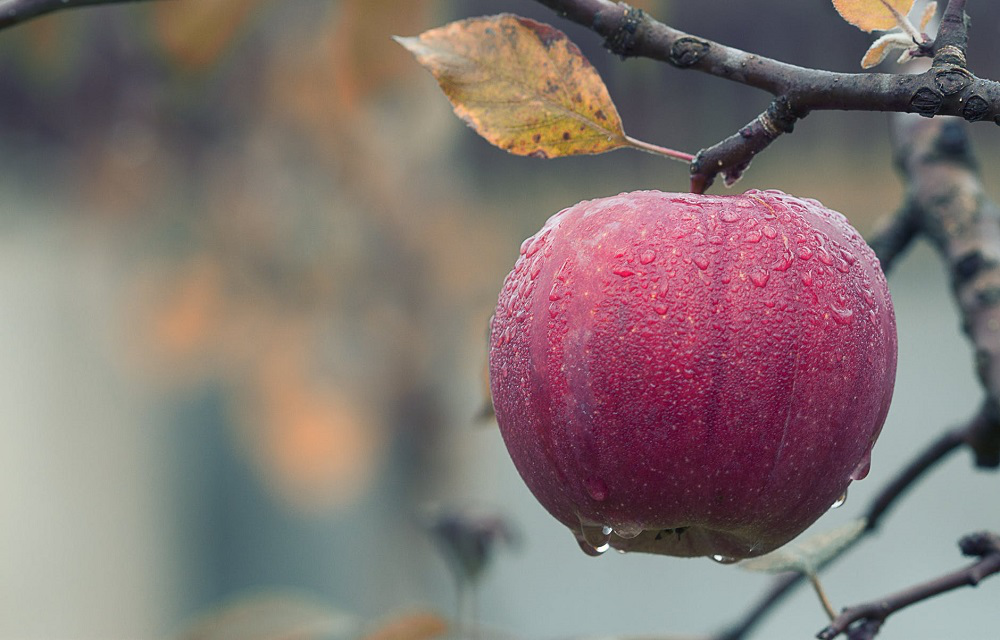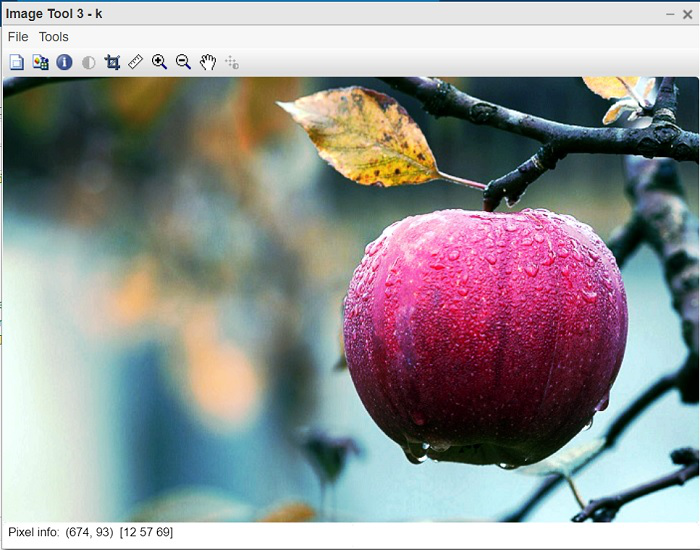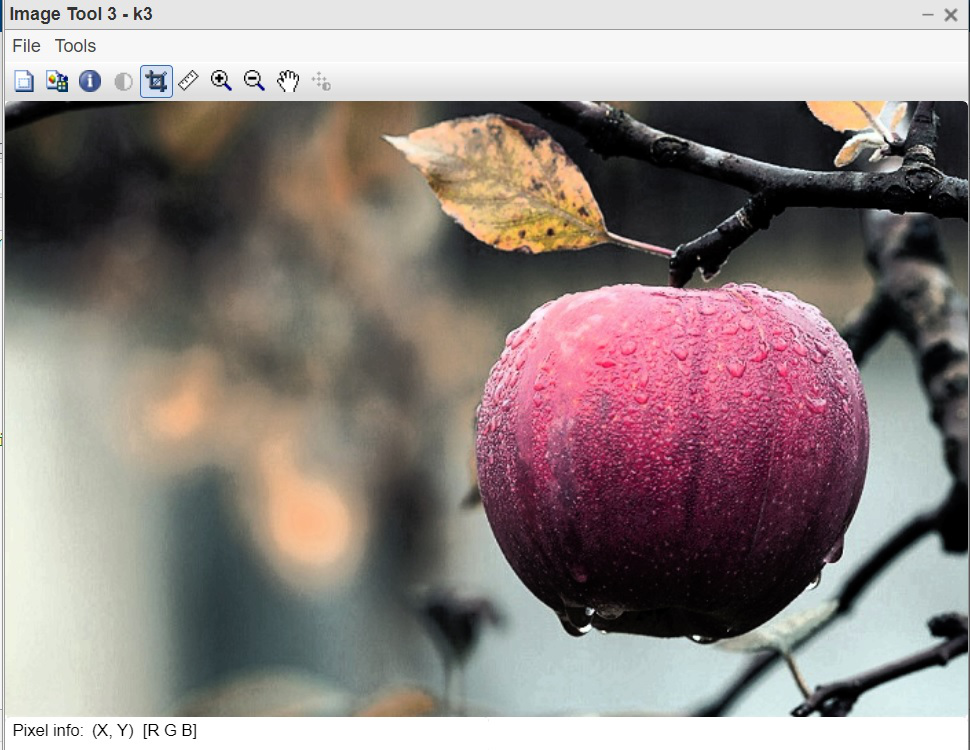# How to Perform Contrast Enhancement of Color Image in MATLAB?

• Difficulty Level : Expert
• Last Updated : 22 Nov, 2021

Colour Images consist of 3 channels each representing particular colour information in RGB format. RGB stands for Red, Green and Blue. All the colours are generated by the proportional mixture of these 3 colours only. When it comes to contrast enhancement, there is a typical dilemma in deciding the appropriate approach for that. One way is to enhance the contrast of each channel separately and the second way is to enhance the grayscale component only without disturbing the real colour component of the image. Both approaches are explained in detail in this article.

There are two approaches for enhancing the contrast of colour images:

• Naive method: Enhance each colour channel separately.
• Standard method: Using HSV colour model.

### Naive algorithm:

Apply contrast enhancement of Red channel, Green channel, and Blue channel separately. As a result, each color component will be enhanced accordingly and the resultant color combination will be very different from the original color combination.

Steps for Naive algorithm:

• Apply histogram equalisation on each channel for RGB separately.
• Show both the images.

Function Used:

• histeq( ) inbuilt function is used to apply histogram equalisation.
• imtool( ) inbuilt function is used to display image.

Example:

## Matlab

 `% MATLAB code for Histogram Equalisation``% on RGB image.``% read the image``k=imread(``"apple.jpeg"``);`` ` `% Display original image.``imtool(k,[]);`` ` `% Apply histogram equalisation.``% on Red channel``k(:,:,1)=histeq(k(:,:,1));`` ` `% on Green channel``k(:,:,2)=histeq(k(:,:,2));`` ` `% on Blue channel``k(:,:,3)=histeq(k(:,:,3));`` ` `% Display enhanced image.``imtool(k,[]);`

Output:Figure 1: Original RGB imageFigure 2: Enhanced image

#### Code Explanation:

• k(:,:,1)=histeq(k(:,:,1)); This line performs contrast enhancement of Red channel.
• k(:,:,2)=histeq(k(:,:,2)); This line performs contrast enhancement of Green channel.
• k(:,:,3)=histeq(k(:,:,3)); This line performs contrast enhancement of Blue channel.
• imtool(k,[]); This line displays enhanced image.

### Standard Algorithm:

First, convert the RGB image into HSV format. H and S channels contain the color information while the V channel contains the brightness value which is similar to grayscale information. The idea is to apply contrast enhancement only of V channel keeping H and S undisturbed,  this way the original color combination will be preserved and as a result, the resultant image will be of high quality.

Steps:

• Convert RGB image into HSV format.
• Apply histogram equalization on the Value channel.
• convert HSV back into RGB format.
• Display resultant RGB image.

Function Used:

• rgb2hsv( ) inbuilt function is used to convert RGB into HSV image.
• histeq( ) inbuilt function is used to apply histogram equalisation.
• hsv2rgb( ) inbuilt function is used to convert HSV into RGB.
• imtool( ) inbuilt function is used to display image.

## Matlab

 `% MATLAB code for apply Histogram ``% Equalisation on HSV image.`` ` `% Read the image.``k1=imread(``"apple.jpeg"``);`` ` `% Display original RGB image.``imtool(k1,[]);`` ` `% Convert RGB into HSV image.``k2=rgb2hsv(k1);`` ` `% Apply HE on Value part of HSV image.``k2(:,:,3)=histeq(k2(:,:,3));`` ` `% Convert HSV to RGB.``k3=hsv2rgb(k2);`` ` `% Display RGB image.``imtool(k3,[]);`

Output:Figure 1: Original RGB imageFigure 2: Enhanced image

Code Explanation:

• k2=rgb2hsv(k1); This line converts RGB input image into HSV format.
• k2(:,:,3)=histeq(k2(:,:,3)); This line applies contrast enhancement on Value part of HSV image.
• k3=hsv2rgb(k2); This line Converts HSV back to RGB.
• imtool(k3,[]); This line displays resultant RGB image.

Histogram equalisation on RGB images alters the colour combination of the final image. The reason is very simple cause it applies equalisation on 3 channels separately. Whereas equalisation on HSV image is performed only on one channel that is Value. The Hue and Saturation channels are not undergoing histogram equalisation therefore, the colour combination is not altering. The second method is preferred over the first method in order to preserve the color combination of the original image into the final enhanced image.

My Personal Notes arrow_drop_up